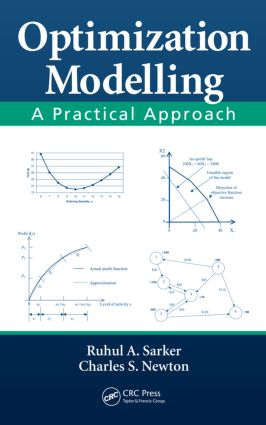Optimization Modelling

A Practical Approach, 1st Edition

CRC Press

502 pages | 96 B/W Illus.

Purchasing Options:\$ = USD
Hardback: 9781420043105
pub: 2007-10-15
SAVE ~\$36.00
\$180.00
\$144.00
x
eBook (VitalSource) : 9780429139109
pub: 2007-10-15
from \$28.98

FREE Standard Shipping!

Description

Although a useful and important tool, the potential of mathematical modelling for decision making is often neglected. Considered an art by many and weird science by some, modelling is not as widely appreciated in problem solving and decision making as perhaps it should be. And although many operations research, management science, and optimization books touch on modelling techniques, the short shrift they usually get in coverage is reflected in their minimal application to problems in the real world. Illustrating the important influence of modelling on the decision making process, Optimization Modelling: A Practical Approach helps you come to grips with a wide range of modelling techniques.

Highlighting the modelling aspects of optimization problems, the authors present the techniques in a clear and straightforward manner, illustrated by examples. They provide and analyze the formulation and modelling of a number of well-known theoretical and practical problems and touch on solution approaches. The book demonstrates the use of optimization packages through the solution of various mathematical models and provides an interpretation of some of those solutions. It presents the practical aspects and difficulties of problem solving and solution implementation and studies a number of practical problems. The book also discusses the use of available software packages in solving optimization models without going into difficult mathematical details and complex solution methodologies.

The emphasis on modelling techniques rather than solution algorithms sets this book apart. It is a single source for a wide range of methods, classic theoretical and practical problems, data collection and input preparation, the use of different optimization software, and practical issues of modelling, model solving, and implementation. The authors draw directly from their experience to provide lessons learned when applying modelling techniques to practical problem solving and implementation difficulties.

Introduction

General Introduction

History of Optimization

Optimization Problems

Mathematical Model

Concept of Optimization

Classification of Optimization Problems

Organization of the Book

References

Exercises

The Process of Optimization

Introduction

Decision Process

Problem Identification and Clarification

Problem Definition

Development of a Mathematical Model

Deriving a Solution

Sensitivity Analysis

Testing the Solution

Implementation

Chapter Summary

Exercises

Introduction to Modelling

Introduction

Components of a Mathematical Model

Simple Examples

Analysing a Problem

Modelling a Simple Problem

Linear Programming Model

More Mathematical Models

Integer Programming

Multi-Objective Problem

Goal Programming

Nonlinear Programming

Chapter Summary

Exercises

MODELLING TECHNIQUES

Simple Modelling Techniques I

Introduction

The Use of Subscripts in Variables

Simple Modelling Techniques

Special Types of LP

Chapter Summary

References

Exercises

Simple Modelling Techniques II

Introduction

Precedence Constraints

Either-or Constraints

K out of N Constraints must Hold

Yes-or-No Decisions

Functions with N Possible Values

Mutually Exclusive Alternatives and Contingent Decisions

Linking Constraints with the Objective Function

Piecewise Linear Functions

Nonlinear to Approximate Functions

Deterministic Models with Probability Terms

Alternate Objective Functions

Constrained to Unconstrained Problem

Simplifying Cross Product of Binary Variables

Fractional Programming

Unrestricted Variables

Changing Constraint and Objective Type

Conditional Constraints

Dual Formulation

Regression Model

Stochastic Programming

Constraint Programming

Chapter Summary

References

Bibliography

Exercises

Modelling Large-Scale and Well-Known Problems I

Introduction

Use of the Summation Sign

Use of the Subset Sign

Network Flow Problems

The Knapsack Problem

Facility Location and Layout

Production Planning and Scheduling

Logistics and Transportation

Chapter Summary

References

Exercises

Modelling Well-Known Problems II

Introduction

Job and Machine Scheduling

Assignment and Routing

Staff Rostering and Scheduling

Scheduling and Timetabling Problem

Chapter Summary

References

Exercises

Alternative Modelling

Introduction

Modelling under Different Assumptions

Hierarchical Modelling: An Introduction

Chapter Summary

References

MODEL SOLVING

Solution Approaches: An Overview

Introduction

Complexity and Complexity Classes

Classical Optimization Techniques

Heuristic Techniques

Optimization Software

Chapter Summary

References

Appendix-9A: LINDO /LINGO

Appendix -9B: MPL

Appendix -9C: GAMS

Appendix -9D: Solver

Appendix -9E: Win QSB

Input Preparation and Model Solving

Introduction

Data and Data Collection

Data Type

Data Preparation

Data Preprocessing

Model Driven Data vs. Data Driven Model

Model Solving

Chapter Summary

References

Exercises

Appendix-10A: Additional Problem Solving using LINGO

Output Analysis and Practical Issues

Introduction

Solutions and Reports

Sensitivity Analysis

Practical Issues and Tips

Risk Analysis

Chapter Summary

Exercises

Basic Optimization Techniques

Introduction

Graphical Method

Simplex Method

Branch and Bound Method

Chapter Summary

References

Exercises

Models For Practical Problems I

Introduction

A Crop Planning Problem

Power Generation Planning

A Water Supply Problem

A Supply Chain Problem

Coal Production and Marketing Plan

General Blending Problem

Chapter Summary

References

Models for Practical Problems II

Introduction

A Combat Logistics Problem

A Lot Sizing Problem

A Joint Lot-Sizing and Transportation Decision Problem

Coal Bank Scheduling

A Scaffolding System

A Gas-Lift Optimization Problem

Multiple Shifts Planning

Chapter Summary

References

Solving Practical Problems

Introduction

A Product-Mix Problem

A Two-Stage Transportation Problem

A Crop Planning Problem

Power Generation Planning Problem

Gas Lift Optimization

Chapter Summary

References

Appendix-A: Crop Planning LP Model